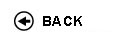Politecnico di Torino
02BNYMQ, 02BNYJM, 02BNYLI, 02BNYLJ, 02BNYLL, 02BNYLM, 02BNYLN, 02BNYLS, 02BNYLU, 02BNYLX, 02BNYLZ, 02BNYMA, 02BNYMB, 02BNYMC, 02BNYMH, 02BNYMK, 02BNYMN, 02BNYMO, 02BNYNX, 02BNYNZ, 02BNYOA, 02BNYOD, 02BNYPC, 02BNYPI, 02BNYPL, 02BNYPM, 02BNYPW
Applied mathematics
1st degree and Bachelor-level of the Bologna process in Mathematics For Engineering - Torino
1st degree and Bachelor-level of the Bologna process in Mechanical Engineering - Torino
1st degree and Bachelor-level of the Bologna process in Automotive Engineering - Torino
Espandi...
 Teacher Status SSD Les Ex Lab Tut Years teaching Delitala Marcello EdoardoO2 MAT/07 40 20 0 0 11
 SSD CFU Activities Area context MAT/07 6 D - A scelta dello studente A scelta dello studente
Esclusioni:
11CWH; 02CWH
 Subject fundamentals The main objectives of the course are to provide knowledge of applied mathematics suitable for the study of differential mathematical models, and the related qualitative and computational analysis by means of appropriate mathematical methods. The course is a natural connection between the basic courses of mathematics and the applied courses of master degree with mathematical foundation. The mathematical modeling is designed to make intelligible, through the rigor of the mathematical formalism, the physical reality or one or more phenomena. These models are developed with specific examples and applications in various engineering fields such as, aerospace (instability flattering and gallopping), environmental (diffusion of a pollutant), biomedical and bioengineering (population dynamics and pattern formation in animals and groups of living entities), civil (beam vibrations, traffic, heat conduction), communication and electronics (dynamic systems of electrical circuits, propagation of a signal and transport equations), physics (models for complex systems). The mathematical methods allow to obtain solutions or make a qualitative analysis of these models thus highlighting the properties, emergent behaviors and phenomena expected. Expected learning outcomes The student will learn deal with differential mathematical models and to qualitatively solve the related mathematical problems. Prerequisites / Assumed knowledge Knowledge of the mathematical methodology obtained with courses of Calculus. Contents Mathematical modeling, representation scales, classification and examples. Mathematical models defined in term of the ordinary differential equations. Solution methods for linear problems. Laplace transform and applications to equations and systems of linear differential equations of second order with constant coefficients. Nonlinear systems. Phase space. Equilibrium configurations. Stability. Linear stability criterion. Nonlinear stability and Lyapunov functional. Bifurcation diagrams. Fork bifurcation, supercritical and subcritical bifurcation. Examples and applications (flattering instability and galloping for aerospace applications, Malthus and logistic for population dynamics, electric circuits RCL and Van der Pol equation) Classification of mathematical models defined in terms of partial differential equations. Diffusion equation, derivation and properties of the solution. Initial value problems and boundary value problems. Solution methods for linear problems and method of separation of variables. Examples and applications (polluting diffusion, heat conduction). Reaction-diffusion equations and applications in biology (morphogenesis and pattern formation) Stationary problem. Laplace equation and elliptic equations (drum). Transport equation and conservation laws. Initial and boundary values problems for linear hyperbolic equations of the first order. Method of characteristics and properties of the solution. Nonlinear hyperbolic equations of the first order. Examples and applications (vehicular traffic, signal transport). Convection-diffusion equations. Wave equation. Initial and boundary values problems for hyperbolic equations of second order. Properties of the solution. Fundamental solution of d'Alembert. Examples and applications in bounded domains (vibrating string and beam). Delivery modes Exercises in classroom will be carried out on these arguments: Laplace transform and solution of linear differential equations Stability and bifurcation of dynamical systems Separation of variables for hyperbolic and parabolic equations Method of characteristics for hyperbolic equations Solution of stationary problems Texts, readings, handouts and other learning resources Notes in Italian available at the �press center�. Further reading: N. Bellomo, E. De Angelis, M. Delitala, Lecture Notes on Mathematical Modelling in Applied Sciences, SIMAI e-Lecture Notes, 1-148, 2008. http://cab.unime.it/journals/index.php/lecture/issue/view/5 N. Bellomo, L. Preziosi, Modelling Mathematical Methods and Scientific Computation, CRC Press, 1995. S. J. Farlow, Partial Differential Equations for Scientists and Engineers, Dover (New York). S. Salsa, F. Vegni, A. Zaretti, P. Zunino, Invito alle Equazioni a Derivate Parziali, Springer Italia, 2009. Assessment and grading criteria The exam consists of a written test. If the student has passed the written with a minimum score of 18, he/she can sustain the oral exam to try to improve the final score of the exam. The written examination consists in solving different exercises to test the ability to apply methods and techniques learned for the qualitative analysis and resolution of problems and differential models. Examples of recurring types of exercises: - Solve, by applying the Laplace transform, the following differential problem. - Given the following dynamic system, determine the equilibrium configurations and their stability. - Solve by the method of characteristics, the following first order PDE transport. - Apply the separation of variables to find the solution of the following PDE. - Determine the stationary solution of the following mathematical problem. The exercises also include a theoretical-conceptual question (without proofs), examples of possible questions are given below. The maximum time available is 120 minutes. During the examination it is allowed to keep only a form consisting of one A4 paper completed by the student . The oral examination consists of two or three questions that include discussion of the written exam and in particular the non-performed or performed incorrectly exercises and some questions about the course program. Examples of possible questions: - Laplace transform and applications. - Dynamical systems: equilibria and linear stability criterion. - Stability of nonlinear dynamic systems. - Bifurcations in dynamical systems. - Classification of mathematical models of partial derivatives. - Mathematical formulation of the mathematical problem. - Diffusion equation and properties of solutions. - Stationary solutions of diffusion equations and elliptic equations. - Separation of variables and applications. - Conservation laws. - Method of characteristics. - Equations of transport and properties of solutions. - Wave equation and properties of solutions. Programma definitivo per l'A.A.2015/16© Politecnico di Torino
Corso Duca degli Abruzzi, 24 - 10129 Torino, ITALY